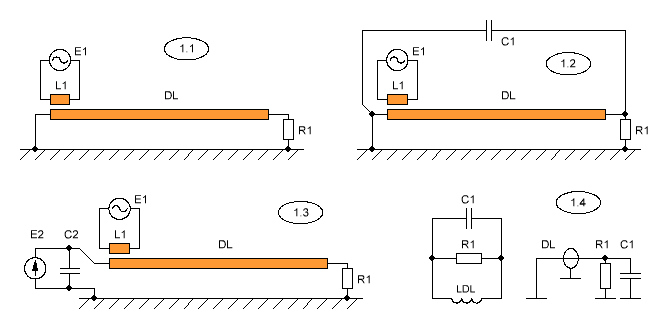Research website of Vyacheslav Gorchilin
2016-12-04
The lumped and distributed resonances in the inductor
Hams and seekers of free energy, there are questions about the different types of resonance that we are here and will try to illuminate. The problem is what it is. Everyone knows that an inductor (hereinafter, simply coil) can be considered as concentrated, and as a long line. In the first case, the formula for determining the resonance will be performing classical Thompson formula: $f = 1/2\pi\sqrt{L\,(C_l + C)}\,, \qquad (1)$ where $$L$$ is the inductance of the coil, $$C_l$$ is its own capacity, and $$C$$ — the external capacity connected in parallel to the coil.
In the second case, we consider the coil as a long line, where the wave takes some time to get from one end to the other. If this time is equal to the oscillation period of the oscillator, in a coil set the mode of the standing wave. In this case, the simplified formula would be: $f = {c\,k \over l}\,, \qquad (2)$ where $$c$$ is the speed of light, $$k$$ — deceleration rate, which, in General, depends on the material of the dielectric, and $$l$$ is the length of the winding wire in the coil. This formula is greatly simplified, since $$f$$ is actually nonlinearly dependent on the length and winding pitch, coil diameter and material of the dielectric. A more accurate formula can be found in . The differences in these two approaches are already visible at this stage, and we will demonstrate that there are many more.

Since the real processes that occur in the coil, is little known, and we shall not stop. Consider the mathematical model which with sufficient accuracy describe these processes, and the results of such calculations can be seen on real devices.

Two models
Consider the direct asymmetric long line DL in figure 1.1, which is excited from a sinusoidal voltage source E1, through the inductor L1. Take her private for the case where DL fits in exactly half the wavelength. Thus, on its edges we get the maximum current, and in the center of the maximum voltage. All measurements will be carried out at a load resistance R1, the value of which will choose a lot less the wave resistance of the line. For the manifestation of two independent processes it is also important that DL was as far away from earth. For the mathematical model this means that if DL is to roll into a coil, its diameter will be much smaller this distance. In this case, the calculation of the line is about to produce by the formula (2), since the parasitic capacitance is practically nonexistent and work well with the classical formula of Telegraph equations.If DL put in parallel to the capacitor C1 (Fig. 1.2), it will begin to work one process, almost do not affect the wave. It is obvious that in this case we are dealing with an oscillatory circuit, the inductance which is the inductance of the line segment DL. I.e. a second, independent model, which can be easily calculated by the classic formula of Thompson (1).
Now it is easy to imagine what happens if we turn this long line in a coil — slightly changes its own capacitance and inductance, and two of the process and will work almost independently from each other. By the way, in this case, the wave model is better to rely on more precise formula, presented in .
Why are these processes, and thus their models, are independent? Look at figure 1.4, which shows their equivalent circuits. DL is in the standing wave, and since R1 is much less than the wave resistance, wave — almost no losses (right). On the wave process capacity C1 does not render almost any influence, but it forms with the inductance DL RLC circuit, which in turn also works independently (left figure). Changing C1 also has no effect on the wave process, but completely changes the picture of the LC resonance.
According to the author, the independence of the processes due to their work in different reference systems. The assemblage point, or point of combining these two systems is the load R1.
For skeptics who believe that in the coil (or in a long line) can't go two independent processes at the same time, a simplified model (Fig. 1.3), which added another DC power source E2. It is bridged by a capacitor C2 to a lower internal resistance at high frequency. As you can see on R1 we get the constant offset from E2, and independent of the antinode of the current standing wave from E1.
The combination of the two models
Next, we will focus on the DL of a rolled coil. This option is currently the best studied. Our great experimenters provided the data, which suggests that when combining two models, the resistance of R1 increases dramatically the amplitude of the signal that rightly can be compared with the increase of the quality factor. This becomes very clear if we consider two independent models: if the processes are combined, it really will be the addition of the amplitudes. Also, researchers say about "the toe" — the phenomenon in which the increasing amplitude is kept even if there is some frequency deviation oscillator. But we'll talk about it another time, but for now imagine a calculatorthat can find points of combining the two models.
In fairness it should be noted that for combining standing wave and LC resonance, working on the harmonics, all the same it is better to use a non-inductive winding DL. Then the wave process is almost unchanged, and focused resonance is easier. This type of winding the researchers called a "grenade" or "dumbbell".
The materials used
1. Alan Payne. SELF-RESONANCE IN COILS, 2014